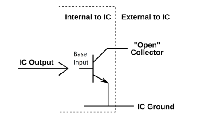Oproep: Ik zoek iemand die kan zorgen voor de continuïteit van deze website

Contents[Show/Hide]

# Handy Arduino functions

Published: 29 February 2020
Last updated: 13 February 2023
Contents[Show/Hide]

### Easy printing debug values in Arduino for Excel

It often happens that I want to show variables in an Excel graph. This can be done very simply, see here:
Simple example

```#include <Streaming.h>
#define _ <<" "<<
int v1=547839, v2=88, v3=9999, v4=0, v5=-301, v6=666;

void setup(void)
{ Serial.begin(115200);
Serial.println("\nval1 val2 val3 val4 val5 val6");
}

void loop(void)
{ delay(100);
Serial << v1 _ v2 _ v3 _ v4 _ v5 _ v6 _ endl;
}```

Turn autoscroll off in the serial monitor and copy the values to Excel with paste special. Than create the chart in Excel.

```int v1=547839, v2=88, v3=9999, v4=0, v5=-301, v6=666;

void setup(void)
{ Serial.begin(115200);
Serial.println("\nval1 val2 val3 val4 val5 val6");
}

void loop(void)
{ delay(100);
// hard way of printing debug values for Excel:
Serial.print(v1);
Serial.print(" ");
Serial.print(v2);
Serial.print(" ");
Serial.print(v3);
Serial.print(" ");
Serial.print(v4);
Serial.print(" ");
Serial.print(v5);
Serial.print(" ");
Serial.println(v6);
}```

There are also extensive debug libraries for the Arduino:
https://randomnerdtutorials.com/serialdebug-library-arduino-ide/

### Serial vs SerialUSB

Most Arduino sketches, which are written for the Arduino Uno, use the Serial.print() to print to the serial monitor. The Arduino Zero, which uses the SAMD21, requires SerialUSB.print() instead, if you want to print to the native USB port.
This little code helps, you can use Serial.print() for the Arduino Zero too. You don't have to modify Arduino sketches Zero anymore for using it on the SAMD21.

```#if defined(__arm__)
#define Serial SerialUSB
#endif```

Maximum loops

Arduino formerly contained a stop button to stop the serial monitor. This button is gone unfortunately, so now you have to pull out the USB plug to stop the Arduino sending continuously data to the serial monitor. With the function maxLoops you can specify the maximum number of loops, so the serial monitor doesn't fills up anymore.

Function

```void maxLoops(const unsigned long loops)
{ static unsigned long i=0;
if(++i > loops) while(1);
}```

Example

```void loop(void)
{ maxLoops(3);
...
...
}```

### SimpleSoftPWM

A PWM output can be created without the use of a hardware timer, which is convenient because the number of timers is limited. The output frequency is just 50 Hz, and it can therefore be used for applications which do not require high-speed PWM, such as LED or heating control.

Example

```const byte LedPin = 12;
SimpleSoftPWM pwm1;

void setup(void)
{
}

void loop(void)
{  digitalWrite(LedPin, pwm1.pwm(180)); // dimmed LED 180/255
}```

Class

```class SimpleSoftPWM // only for LEDs and heating
{ public:
bool pwm(byte value); // 0 ... 255

private:
unsigned long start_us, periodTime_us=19890; // minimum frequency to prevent LED flickering = 50Hz
};```

```bool SimpleSoftPWM::pwm(byte value) // 0 ... 255, only for LEDs
{ unsigned long now_us = micros();
if((now_us - start_us) >= periodTime_us) start_us = now_us;
return((now_us - start_us) < value * 78); // 19890/255=78
}```

### Arduino open drain outputThe Arduino has no open drain or open collector outputs but these are easy to create by software. When the output is high, the impedance must be high; this is done by making the pinmode INPUT. When the output is low, it must be 0V; this is done by making the pinmode OUTPUT and the output level LOW.

Example

`openDrain(byte pin, bool value);`

Function

```void openDrain(byte pin, bool value)
{ if(value) pinMode(pin, INPUT);
else pinMode(pin, OUTPUT);
digitalWrite(pin, LOW);
}```

### Using a macro to determine the length of an array

Generally, a constant value is used to hold the size of an array. However, this is risky. The value can be wrong, which cause nasty bugs:

```const int wattTable[] = {0, 125, 250};
for(int i=0; i<3; i++) // this is risky
{
} ```

It is safer to calculate the array size with sizeof instead of using a constant. Because the calculation is done during compile-time, no extra code is generated.

```#define arrayLenght(array) sizeof(array)/sizeof(array) // compile-time calculation
for(int i=0; i<arrayLenght(wattTable); i++)
{
}```